# NCERT Solutions for Class 11 Chemistry Chapter 8 Redox Reactions

NCERT solutions for class 11 chemistry chapter 8 Redox Reactions- Solutions of NCERT class 11 chapter 8 Redox Reactions discusses the concept of reduction and oxidation in detail and various insights about the redox reaction. Also, it discusses oxidation number, balancing redox reactions, types of a redox reaction, loss and gain of electrons by the elements in the reaction, change in oxidation number and applications of redox reactions. In CBSE NCERT solutions for class 11 chemistry chapter 8 Redox Reactions, there are 30 questions in the exercise. The solutions of NCERT class 11 chemistry chapter 8 Redox Reactions are prepared and designed by experts. Chemistry is a scoring subject for competitive exams. These NCERT solutions for class 11 chemistry chapter 8 Redox Reactions will help you in your preparation of class 11 final examination as well as in the various competitive exams like JEE, NEET etc. If you are looking for an answer from any other chapter even from any other class then go with NCERT Solutions, there you will get all the answers of NCERT easily.

Redox reactions are the class of reactions in which reduction and oxidation occur simultaneously. In NCERT solutions for class 11 chemistry chapter 8 Redox Reactions you will get to know three-tier conceptualization of redox reactions which are classical, electronic, and oxidation number. Two methods, oxidation number, and half reaction method are used to balance chemical equations for redox reactions. Oxidation number method is based on the change in the oxidation number of oxidizing agent and the reducing agent and in half-reaction method, redox reaction split into two half reactions-one involving reduction and the other involving oxidation.

After completing NCERT solutions for class 11 chemistry chapter 8 Redox Reactions you will be able to identify redox reaction; define terms like reduction, oxidation, reducing agent and oxidising agent; explain mechanism of redox reaction by electron transfer process; identify reductant and oxidation by using the concept of  oxidation number; balance chemical equations by using two methods-oxidation number method and half reaction method.

## Important points of Class 11 Chemistry Chapter 8 Redox Reactions-

1. Redox reactions are the class of reactions in which reduction and oxidation occur simultaneously.

2.  The oxidation number represents the total number of electrons gained or lost by an atom.

3. Oxidation is the process which involves the addition of oxygen or removal of hydrogen.

4. The reduction is the process which involves removal of oxygen or addition of hydrogen.

## Topics of NCERT Grade 11 Chemistry Chapter 8 Redox Reactions

8.1 Classical Idea of Redox Reactions-Oxidation and Reduction Reactions

8.2 Redox Reactions in Terms of Electron Transfer Reactions

8.3 Oxidation Number

8.4 Redox Reactions and Electrode Processes

## NCERT Solutions for Class 11 Chemistry Chapter 8 Redox Reactions - Exercise Questions

solution-

O.N is the oxidation number

O.N of Oxygen() = -2 ( In case of peroxide and superoxide it wil be different ON)

O.N of hydrogen()= +1 (In case of metalic hydride, -1)

O.N of sodium () = +1

O.N of aluminium () = +3

O.N of potassium ()= +1

O.N of calcium () = +2

In neutral compounds the sum of O.N of all the atoms is zero.

(a) Let the O.N of P be x

(b) Let the O.N of S be x

(c)  Let the O.N of P be x

(d) Let the O.N of Mn be x

(e) Let the O.N of O be x

Ca is an alkaline earth metal so its O.N. is +2

(f) Let the O.N of B be x

Note that in this H exists as hydride ion   so its O.N. is -1

(g) Let the O.N of S be x

(h) Let the O.N of S be x

Solution-

O.N of potassium ()= +1

O.N of hydrogen()= +1 (In case of metalic hydride, -1)

O.N of Oxygen() = -2 ( In case of peroxide and superoxide it wil be different ON)

1*1 + 3*x = 0

x = (-1/3)

average  O. N. Of is    . But it is wrong because  O.N cannot be fractional. So lets try with structure of

O.N of  = -1 (because a coordinate bond is formed between   and    ion. Hence O. N of three atoms are 0,0 and -1, O.N 0 in moleculeand -1 in  ion.)

Assume O.N of S is x

Fractional O.N  is not possible so try with structure -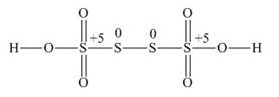The two S atom present in the middle has zero (0) O.N and other two have (+5) O.N [ -2 from two O atom and -1 from OH]

If you calculate the oxidation number of Fe in  it would be 8/3 and however, O.N cannot be in fractional.

Here one iron atom has +2 O.N and the other two are of +3 O.N.

let assume carbon has x oxidation Number

So,[ x + 1(3) +x +1(2) +(-2)+1 = 0]

2x = -4

x=-2

In this molecule two carbon atoms present in different enviroments. Hence, they cannot have the same O.N.Thus, C exhibits the O. S  of -3 and -1.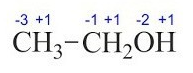suppose the oxidation number of Carbon is x.

If we calculate the O.N of x we get x=0

However, 0 is average O.N. of C atoms. In this molecule two carbon atoms present in different enviroments. Hence, they cannot have the same O.N.Thus, C exhibits the O.S  of +3 and –3 in CH3COOH This can be more understood by structure-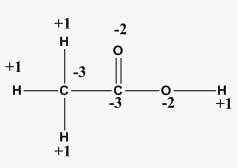Here we can see that at right C, +3 O.N (-1 from OH and -2from O atom) and in left C, -3 O.N(contribution from H atom only)

Question

Solution-

Let us write the O.N of each element

Here, the O.N of Cu decreases from +2 to 0 i.e., CuO is reduced to Cu. Also, the O.N of H increases from 0 to +1 i.e., H2 is oxidized to H2O. Hence it is a redox reaction.

Question

Solution-

Let us write the O.N of each element

Here, the O.N of Fe decreases from +3 to 0. Also, the O.N of C increases from +2 to +4 . Hence it is a redox reaction.

Question

Solution-

Let us write the O.N of each element

Here, the O.N of Fe decreases from +3 in BCl3  to –3 in B2H6. And, the O.N of H increases from –1 in  to +1 in  . Hence it is a redox reaction.

Question

Solution-

We know that oxidation = loosing of  by atom

and reduction = gaining of  by another atom

here lose its electron and  accept it, Hence it is a redox reaction

Question

Solution-

here  oxidation reaction

and  reduction reaction (oxidation state of oxygen is zero at molecular state )

hence it's a redox reaction

Justify that this reaction is a redox reaction

Solution-

Oxidation state of

Here is oxidized and reduced as well. So, it is a redox reaction.

(i)  let the oxidation number of sulphur be x

So,

There is a fallacy Sulphur cannot have +8 oxidation state because it has maximum +6 oxidation number, not more than that.The structure of is shown as follows: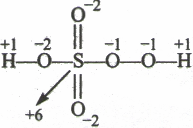(ii)

let the oxidation number of chromium be x

now

There is no fallacy here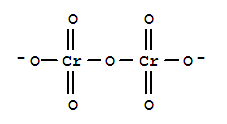(iii)

let assume oxidation number of N is x

Now,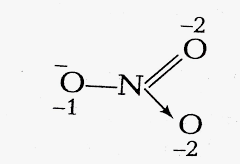here is  no fallacy about the O.N of N in

Question

in this formula, we can see that mercury has  oxidation state

Question

sulphate has  oxidation state

Question

Tin(IV) oxide

Oxygen has  oxidation state

Question

Thallium(I) sulphate

Question

Iron(III) sulphate

Answer- Formula of the compounds:  Iron(III) sulphate is

Question

Answer- Formula of the Chromium(III) oxide compounds is

the substance of Carbon -

Substance

O.N. Of C

-4

-3

-2

-1

0

+1

+2

+3

+4

substance for Nitrogen-

Substance

O.N. Of N

-3

-2

-1

0

+1

+2

+3

+4

+5

• sulphur dioxide  here oxidation state of sulphur is +4  and the range of oxidation number of S is from -2 to +6. It means it can accept an electron and lose as well, therefore, it can behave as oxidant and reductant both.

• In case of hydrogen peroxide  oxidation state is -1 and the oxidation state of O can vary from 0 to -2. So it shows both oxidizing and reducing properties.

• For  , Nitrogen has +5 oxidation state and it varies from +5 to -3. So it only accepts electrons. The oxidation state of N only decreases. Hence it acts as only oxidants.

• And in case of  , the oxidation state of O is zero(0) and the range of oxidtion number of O is 0 to -2. It only decreases in this case also so therefore it acts as only oxidants.

Question

Why it is more appropriate to write these reactions as :

Also, suggest a technique to investigate the path of the above (a) and (b) redox reactions.

(a) In the photosynthesis process-

step 1- the    liberation of   and    -->

step-2 The  produced in above reduces the  into glucose and water

So, the final net reaction is

It is more appropriate to write the reaction as above because water molecule also produced in photosynthesis reaction.

The path of reaction can be investigated by using the radioactive  instead of

(b)

(the final net reaction)

Dioxygen is produced from both steps, one from the decomposition of ozone () and other is from the reaction of hydrogen peroxide with(O)

• The path of the reaction can be investigated by using .

These can be understood by the following examples-

•   is reducing agent and    is an oxidizing agent

[O.N of phosphorus +5]      Higher O.S of P

[O.N of phosphorus +3]     Lower O.S of P

•   is an  is a reducing agent and  oxidising agent

(O is in excess)    [O.N of C +4]

(C is in excess)       [O.N of C +2]

•  is a reducing agent and  is an oxidizing agent

(K is in excess)  [O.N of O -2] (lower O.S.)

(O is in excess) [O.N of O -1] (lower O.S.)

Question

Though alkaline potassium permanganate and acidic potassium permanganate both are used as oxidants, yet in the manufacture of benzoic acid from toluene we use alcoholic potassium permanganate as an oxidant. Why ? Write a balanced redox equation for the reaction.

Alcohol and  both are polar in nature and alcohol is homogenous to toluene because both are organic compounds. So the reaction is faster in the homogenous medium rather than heterogeneous medium. And hence all the compounds react at a faster rate.

Chemical equation-

Question

When concentrated sulphuric acid is added to an inorganic mixture containing chloride, we get colourless pungent smelling gas HCl, but if the mixture contains bromide then we get red vapour of bromine. Why ?

• Concentrated sulphuric acid is added to an inorganic mixture containing chloride-

HCl is a weak reducing agent and it cannot reduce  to   thats why we get colourless pungent smelling gas HCl.

• Concentrated sulphuric acid is added to an inorganic mixture containing bromide-

When conc. sulphuric acid is added to an inorganic mixture containing bromide initially it produces  and it is a strong reducing agent so it reduces  to   with evolution of is a red vapor of bromine.

Substance reduced/oxidizing agent-

Substance oxidized/reducing agent-

Substance reduced/oxidising agent-

Substance oxidised/reducing agent-

Substance oxidized/reducing agent-

Substance reduced/oxidizing agent-

Substance oxidized/reducing agent-

Substance reduced/oxidizing agent-

Question

oxidizing power order

Bromine is a stronger oxidizing agent than iodine. So in the case of bromine (avg. oxidation number of sulphur is changed from +2 to +6)

and in case of iodine it (+2 to +2.5). So that's why thiosulphate reacts differently with bromine and iodine.

part(i)

Fluorine can oxidize other halogen ions. On the other hand   cannot oxidize

And hence we say that fluorine is the better oxidant among halogen.

part(ii)

& are able to reduce  but  are unable to reduce sulphuric acid.

So here we can say that  & are better reductant than .

Again  can only able to reduce  but  cannot.

Hence among hydrohalic compound hydroiodic acid is the best reductant.

Question

What conclusion about the compound    (of which is a part) can be drawn from the reaction ?

we conclude that the oxidation state of Xenon changes from  +8 to +6

and oxidation state of F changes from -1 to 0

is reduced by accepting an electron.

It is a strong oxidizing agent than F

Question 8.17(a)  Consider the reactions:

(a)

What inference do you draw about the behaviour of  and  from these reactions ?

In the first reaction, we can see that   oxidizes the phosphorus from (+1  +5) also in second, we clearly see that   oxidize the phosphorus from (+1+5).

Both are oxidizing agents.

Question 8.17(b)  Consider the reactions:

No change observed

Here, by looking at the reaction, we conclude that   oxidises  and in the second reaction  not able to oxidise. So we can say that is stronger oxidizing agent than .

Question

(In basic medium)

reduction half reaction

(+7 to +4)

Add 3 electron on LHS side and after that to balance charge add OH ions. And to balance O atom add water molecule on whichever side it needed

balance it

oxidation half

balance it

equalising the no. of electrons by multiplying the oxidation half by 3 and reduction half by 2 and then add it.

Question

(In Acidic medium)

oxidation half reaction

reduction half reaction

Balancing the reaction

multiply the oxidation half by 5 and reduction half by 2 and then add these two reactions

Question

(c)

in acidic medium

In acidic medium

oxidation half reaction-

reduction half reaction-

Balancing the reaction

multiply by 2 on oxidation half-reaction then add it with reduction half reaction

Question

in acidic medium

Half-reaction

oxidation half

reduction half

balancing them by multiplying oxidation half by 3 and adding the reaction

Carbon shows different oxidation state according to the compound formula.

here we can clearly say that Carbon is in its +3 oxidation state.

The oxidation state of carbon is increased(oxidized) and decreased(reduced) as well in the product side. So it is a redox reaction and more specifically we can say it disproportion redox reaction.

The base equation

write oxidation half with their oxidation state

Balance the charge on  by adding 1  on RHS side. To balance charge add  ions on RHS side and then for oxygen balance add  molecule on LHS side.

reduction half

balancing the reduction half by adding 1 on LHS side

Add both balanced reduction half and oxidation half

(b) Identify the element that exhibits only positive oxidation state

(c) Identify the element that exhibits both positive and negative oxidation states

(d) Identify the element which exhibits neither the negative nor does the positive oxidation state.

(a) (because of highly electronegative in nature)

(b) (highly electropositive)

(c)(has emplty d orbitals)

(d)  (enert gas

Base equation-  --------------(have to remember)

Now we have to balance the oxidation half and reduction half.

oxidation half

balancing-  Oxygen is balanced by adding water molecule, Hydrogen is balanced by  ion and for charge add (electron)

Reduction hallf

Balancing-  to balance charge add an electron

Now add both balanced oxidation half and reduction half,  we get

(a) Select the possible non-metals that can show disproportionation reaction.

(b) Select three metals that can show disproportionation reaction

(a) Phosphorus, sulphur and chlorine can show disproportionation reaction.

(b) Manganese, copper, indium and gallium can show disproportionation reaction.

we have,

number of moles(n) = given mass/ molecular mass   ------------------------------(eq.1)

No. of moles of ammonia  = 10/17 = 0.588

No. of moles of oxygen  = 20/32= 0.625

Balanced Reaction

Here we see that 4 moles of ammonia required 5 moles of oxygen. So

0.588 moles of ammonia =  moles of  . But we have only 0.625 moles of .

It means oxygen is a limiting reagent and the maximum weight of nitric oxide can be produced by 0.635 moles of

So, 5 moles of  produced 4 moles of C.

therefore 0.625 moles of  =  moles of .

from Eq. 1

mass of  = number of moles * molecular weight

=

= 15 g

Alternate Method

directly consider the molecular weight

(17*4) g of NH3 required (5*32) g of O to produce (30*4) g of NO

So, 10g of NH3 required=  (5*32/17*4)*10 = 23.5g of O. But we have only 20g (means O is limiting reagent) whatever the max. NO produce is from 20g of O.

and we know that 5*32g of O produce 30*4 g of NO

So, 20g of O produce =(30*4/5*32)*20 g of NO = 15g of NO

(a)  and
(b)  and Cu(s)
(c) (aq) and Cu(s)
(d) Ag(s) and  (aq)
(e)  and  .

If  for the overall reaction is positive  feasible

negativenot feasible

(a)

---------------------------------------------------------------------------------------

(b)

---------------------------------------------------------------------------------------

(c)

-------------------------------------------------------------------------

(d)

------------------------------------------------------------------------------

(e)

-------------------------------------------------------------------------------

Question

(i) An aqueous solution of  with silver electrodes

(i)  dissociate into  and

@ Cathode (reduction potential of silver is higher than

@Anode -  (oxidation potential of silver is higher than water molecule.So silver electrode oxidized )

Question

An aqueous solution  with platinum electrodes

(ii) since platinum electrode cannot easily oxidize. So at the anode    will oxidize and liberate oxygen and at cathode   will be deposited.

At cathode-

At anode-

Question

(iii) A dilute solution of  with platinum electrodes

given sulphuric acid is dilute.

ionize into

At cathode

At anode, There will be -(liberation of oxygen gas)

Question

An aqueous solution of CuCl2 with platinum electrodes

(iv) In aqueous solution  ionise into  and

At the cathode, the copper ion will be deposited because it has a higher reduction potential than the water molecule

At the anode, the lower electrode potential value will be preferred but due to overpotential of oxygen, chloride ion gets oxidized at the anode.

@ Anode-

@ cathode-

In order to displace a metal from its metal salt is done only when the other metal has higher electrode potential.

Question

arrange these metals in their increasing order of reducing power.

A negative electrode potential means redox couple is a stronger reducing agent. So as per data the increasing order of the following is-

Question

takes place, Further show:

(i) which of the electrode is negatively charged,

(ii) the carriers of the current in the cell, and

(iii) individual reaction at each electrode.

(i)  electrode is negatively charged because it loses electrons (act as an anode)

(ii) electron flow from negatively charged electrode to a positively charged electrode (anode to cathode) and the flow of current is just reversed. So current flow through silver cathode to the zinc anode.

(iii) At Anode-

At Cathode

## NCERT solutions for class 11 chemistry

 Chapter 1 NCERT solutions for class 11 chemistry chapter 1 Some Basic Concepts of Chemistry Chapter-2 CBSE NCERT solutions for class 11 chemistry chapter 2 Structure of Atom Chapter-3 Solutions of NCERT class 11 chemistry chapter 3 Classification of Elements and Periodicity in Properties Chapter-4 NCERT solutions for class 11 chemistry chapter 4 Chemical Bonding and Molecular Structure Chapter-5 CBSE NCERT solutions for class 11 chemistry chapter 5 States of Matter Chapter-6 Solutions of NCERT class 11 chemistry chapter 6 Thermodynamics Chapter-7 NCERT solutions for class 11 chemistry chapter 7 Equilibrium Chapter-8 CBSE NCERT solutions for class 11 chemistry chapter 8 Redox Reaction Chapter-9 Solutions of NCERT class 11 chemistry chapter 9 Hydrogen Chapter-10 NCERT solutions for class 11 chemistry chapter 10 The S-Block Elements Chapter-11 CBSE NCERT solutions for class 11 chemistry chapter 11 The P-Block Elements Chapter-12 Solutions of NCERT class 11 chemistry chapter 12 Organic chemistry- some basic principles and techniques Chapter-13 NCERT solutions for class 11 chemistry chapter 13 Hydrocarbons Chapter-14 CBSE NCERT solutions for class 11 chemistry chapter 14 Environmental Chemistry

NCERT solutions for class 11 subject wise

 NCERT solutions for class 11 biology Solutions of NCERT class 11 maths CBSE NCERT solutions for class 11 chemistry NCERT solutions for class 11 physics

## Benefits of NCERT solutions for class 11 chemistry chapter 8 Redox Reactions

• The comprehensive answers given in the NCERT solutions for class 11 chemistry chapter 8 Redox Reactions will help you to understand the chapter easily.

• Revision will be a lot much easier such that you always remember the concepts and get very good marks in your class.

• Homework will be easy now, all you need to do is check the detailed CBSE NCERT solutions for class 11 chemistry chapter 8 Redox Reactions and you are good to go.

If you have a doubt or question that is not available here or in any of the chapters, contact us. You will get all the answers that will help you score well in your exams.